Publication date: 07/30/2020

## Control Limits for P and NP Charts

The lower and upper control limits, LCL, and UCL, respectively, are computed as:

P chart LCL =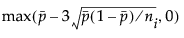P chart UCL =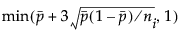NP chart LCL =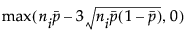NP chart UCL =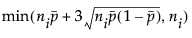where: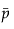is the average proportion of nonconforming items taken across subgroups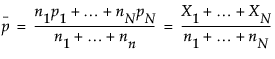ni is the number of items in the ith subgroup